# KS1 Maths Quiz - Year 1 Numbers - Picture Problems (Questions)

This quiz addresses the requirements of the National Curriculum KS1 Maths and Numeracy for children aged 5 and 6 in year 1. Specifically this quiz is aimed at the section dealing with solving problems using objects and pictorial representations.

Picture problems are number questions which use representative illustrations to help solve them. These representations can be useful when counting on or back, as it’s often easier to work out the answer to a problem if you can see a picture of what you’re counting!

1.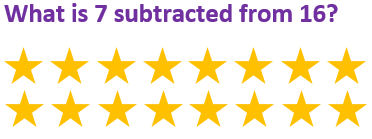[ ] 8 [ ] 9 [ ] 7 [ ] 17
2.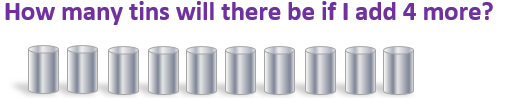[ ] 18 [ ] 15 [ ] 17 [ ] 14
3.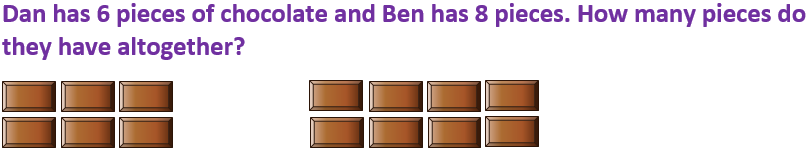[ ] 14 [ ] 15 [ ] 16 [ ] 12
4.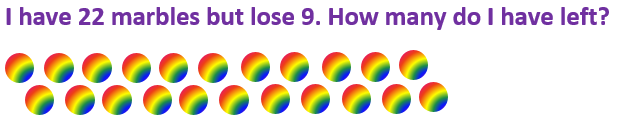[ ] 3 [ ] 13 [ ] 26 [ ] 31
5.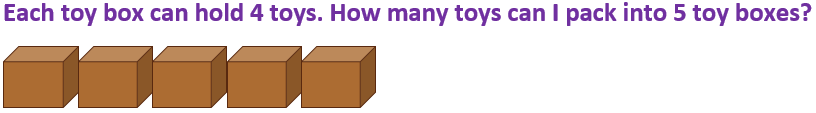[ ] 16 [ ] 14 [ ] 22 [ ] 20
6.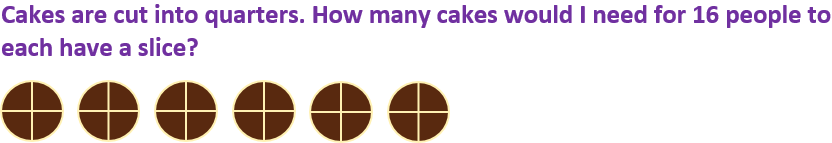[ ] 4 [ ] 6 [ ] 5 [ ] 10
7.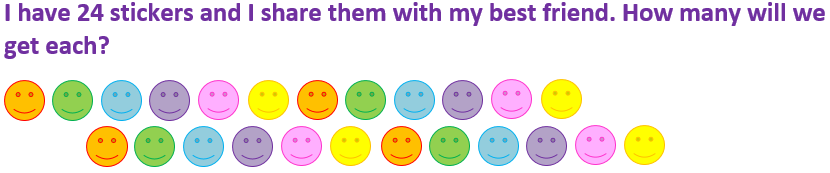[ ] 14 [ ] 10 [ ] 6 [ ] 12
8.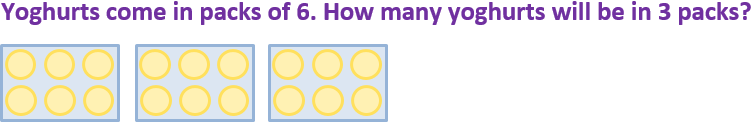[ ] 20 [ ] 16 [ ] 18 [ ] 14
9.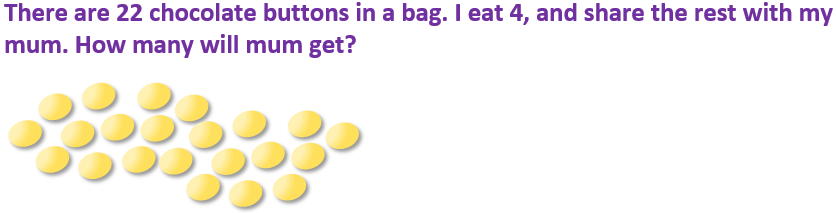[ ] 11 [ ] 8 [ ] 10 [ ] 9
10.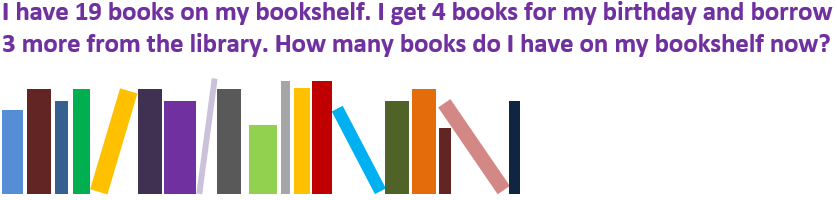[ ] 25 [ ] 28 [ ] 24 [ ] 26KS1 Maths Quiz - Year 1 Numbers - Picture Problems (Answers)
1.[ ] 8 [x] 9 [ ] 7 [ ] 17
If we take 7 away from the 16 stars, 9 are left
2.[ ] 18 [ ] 15 [ ] 17 [x] 14
4 more than 10 is 14
3.[x] 14 [ ] 15 [ ] 16 [ ] 12
6 plus 8 is 14
4.[ ] 3 [x] 13 [ ] 26 [ ] 31
9 subtracted from 22 is 13
5.[ ] 16 [ ] 14 [ ] 22 [x] 20
5 groups of 4 make 20
6.[x] 4 [ ] 6 [ ] 5 [ ] 10
There are 4 groups of 4 in 16
7.[ ] 14 [ ] 10 [ ] 6 [x] 12
Half of 24 is 12
8.[ ] 20 [ ] 16 [x] 18 [ ] 14
3 groups of 6, or 6 + 6 + 6, is 18
9.[ ] 11 [ ] 8 [ ] 10 [x] 9
22 – 4 = 18. Half of 18 is 9
10.[ ] 25 [ ] 28 [ ] 24 [x] 26
19 plus 4 plus 3 is 26